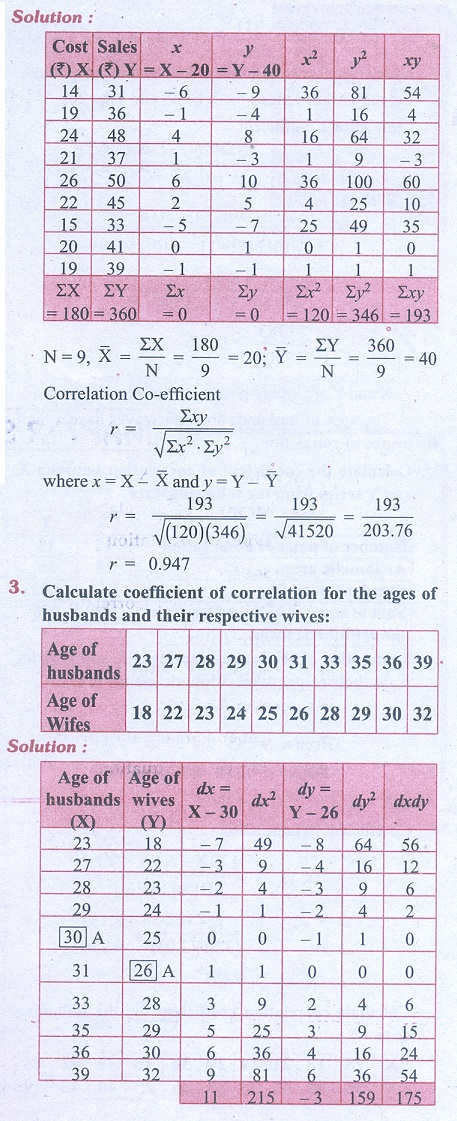Home | | Business Maths 11th std | Exercise 9.1: Spearmanâ€™s Rank Correlation Coefficient

# Exercise 9.1: Spearmanâ€™s Rank Correlation Coefficient

Business Mathematics and Statistics Book back answers and solution for Exercise questions - Statistics: Correlation and Regression analysis : Spearmanâ€™s Rank Correlation CoefficientTags : Problem Questions with Answer, Solution | Correlation and Regression analysis | Statistics , 11th Business Mathematics and Statistics(EMS) : Chapter 9 : Correlation and Regression analysis
Study Material, Lecturing Notes, Assignment, Reference, Wiki description explanation, brief detail
11th Business Mathematics and Statistics(EMS) : Chapter 9 : Correlation and Regression analysis : Exercise 9.1: Spearmanâ€™s Rank Correlation Coefficient | Problem Questions with Answer, Solution | Correlation and Regression analysis | Statistics